### Home > CCA2 > Chapter Ch11 > Lesson 11.2.4 > Problem11-80

11-80.
1. Solve each equation below for x. Show all of your work. (Your answers will contain the variables a, b, and/or c.) Homework Help ✎

1. cxa = b

2.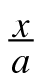b = c

3. (xa)(xb) = 0

4. ax2acx = 0

5.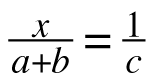6.+ a = b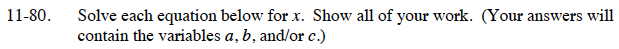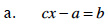$x=\frac{a+b}{c}$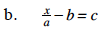x = ab + ac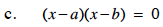x = a or x = b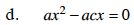Factor and use the Zero Product Property.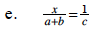Multiply both sides by c(a + b).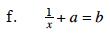Subtract a from both sides and take the reciprocal of both sides.